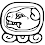## 25 December 2009

### SharpTestsEx 1.0.0Beta : Satisfy your test

More than few improvements, regarding some assertions and its failure message, and some internal refactoring, the mayor change is about the satisfier.

To talk about the syntax of the satisfier is something hard basically because there is no syntax. From user side of view, using the satisfier, the assertion is a simple and pure Func<TA, bool>.

From our side of view, the satisfier is the challenge of show an understandable failure message and, perhaps, help you to understand own much readable and self-explained is the name of some method.

Let me show you some example to understand how the satisfier works.

#### Example 1

Given this empty class

`public class Session{   public bool IsOpen { get; private set; }   public void Open() {}   public void Close() {}}`

The test to pass is:

`var session = new Session();session.Satisfy(s => !s.IsOpen);session.Open();session.Satisfy(s => s.IsOpen);session.Close();session.Satisfy(s => !s.IsOpen);`

The test fail with this message:

SharpTestsExExamples.Session Should Satisfy (s => s.IsOpen)

Implementing the Open method the failure message is:

SharpTestsExExamples.Session Should Satisfy (s => !(s.IsOpen))

#### Example 2 (using Enumerable and LINQ)

In this case the value under test is a IEnumerable<int>:

`var ints = new[] { 1, 2, 3 };`
##### With
`ints.All(x => x.Satisfy(a => a < 3));`

The failure message is : 3 Should Satisfy (a => a < 3)

As you can see the extension Satisfy can be used even as a predicate inside a LINQ expression and, in this way, only the value breaking the test will be showed.

##### With
`ints.Satisfy(a => a.All(x => x < 3));`

The failure message is : [1, 2, 3] Should Satisfy (a => a.All(x => x < 3))

##### The same result using Any
`ints.Any(x => x.Satisfy(a => a > 5));`

The failure message : 1 Should Satisfy (a => a > 5)

#### Example 3 (method call)

`var actual = "sometXing";actual.Satisfy(a => a.ToUpperInvariant().Contains("TH"));`

Fail with : "sometXing" Should Satisfy (a => a.ToUpperInvariant().Contains("TH"))

#### Example 4 (LINQ method call)

`var ints = new[] { 1, 2, 3 };ints.Satisfy(a => a.SequenceEqual(new[] { 3, 2, 1 }));`

As you know SequenceEqual is a LINQ extension method included in .NET 3.5 and the satisfier will recognize it and will show:

[1, 2, 3] Should Satisfy (a => a.SequenceEqual(new[] {3, 2, 1}))
Values differ at position 0.
Expected: 3
Found : 1

#### Example 5 (equal)

`var amount = 135.25m;amount.Satisfy(a => a == 135m);`

Fail with: 135,25 Should Satisfy (a => a == 135)

but

`var name = "ErmAnegildo";name.Satisfy(a => a == "Ermenegildo");`

Fail with :

"ErmAnegildo" Should Satisfy (a => a == "Ermenegildo")
Strings differ at position 4.
ErmAnegildo
Ermenegildo
___^_______

#### Example 6 (complex predicate)

`var actual = "somexxing";actual.Satisfy(a => a.StartsWith("some") && a.Contains("TH") && a.EndsWith("ing"));`

As you can see the assertion is composed by three conditions where only one will fail. The failure message will be:

"somexxing" Should Satisfy (a => a.Contains("TH"))

The same assertion but using “somexxING”, as actual value, will fail with:

"somexxING" Should Satisfy (a => a.Contains("TH"))
And
"somexxING" Should Satisfy (a => a.EndsWith("ing"))

Another example using a more “real” case:

`var users = new UsersRepository();users.Where(u => u.IsActive)   .Satisfy(a => a.Count() == 3 && a.Any(u => u.Name == "John") && a.Any(u => u.Name == "Fabio"));`

The class UsersRepository is a IRepository<User> and the User class implements ToString returning the Name property. The repository contains three active users and does not contain an active User named Fabio. The failure message is:

[John, Frank, Marcus] Should Satisfy (a => a.Any(u => u.Name == "Fabio"))

#### Conclusions

If you are involved in various projects where one use MsTests another use xUnit another use NUnit you don’t need to remember which is the name of the Assertion class nor the syntax of the assertion itself, nor if the first parameter is the expected or the actual… what you need is only write “.Satisfy(” and continue writing pure C# predicate.

Satisfy your test with Sharp Tests Ex.

1.Wow, this looks really nice!

Does it work with MbUnit also?

2.Yes it works. What you need for MbUnit is only the ShapTestsEx.dll.

3.Ok thank you! Going to try this out soon...

4.Hi. Way I can't check a property of tested object in Satisfy? This throws an error "none of the operands includes the value under test.."
e.g.: Account.Satisfy(a => a.Total == Money.EUR(30000));

5.what you are testing there is: Account.Total